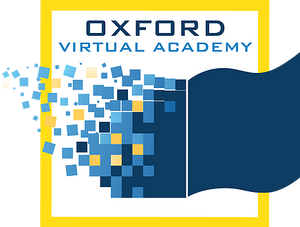# Algebra II1

In Algebra II, students analyze situations verbally, numerically, graphically, and symbolically. Students become proficient at solving equations and inequalities. They extend their knowledge of algebraic expressions, absolute value, functions, and graphs. In this course, students solve equations, inequalities, systems and problems using matrices, inverse matrices, matrix operations, and determinants. Students also learn about different functions and are introduced to the imaginary number i and find complex solutions to equations. Additionally, introduces exponential and logarithmic functions, conic sections, probability, statistics, sequences, and series.

* Prerequisites: Algebra I